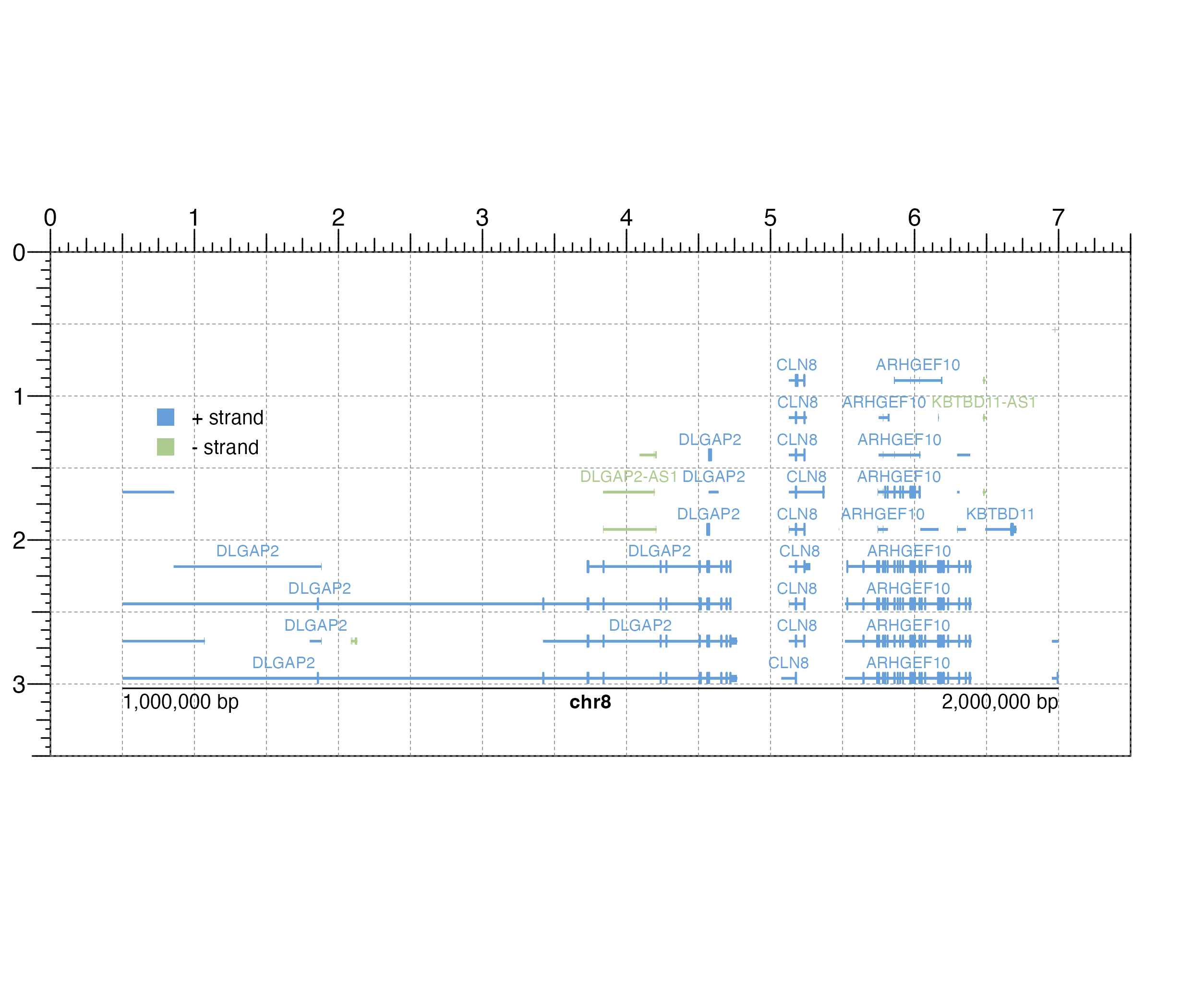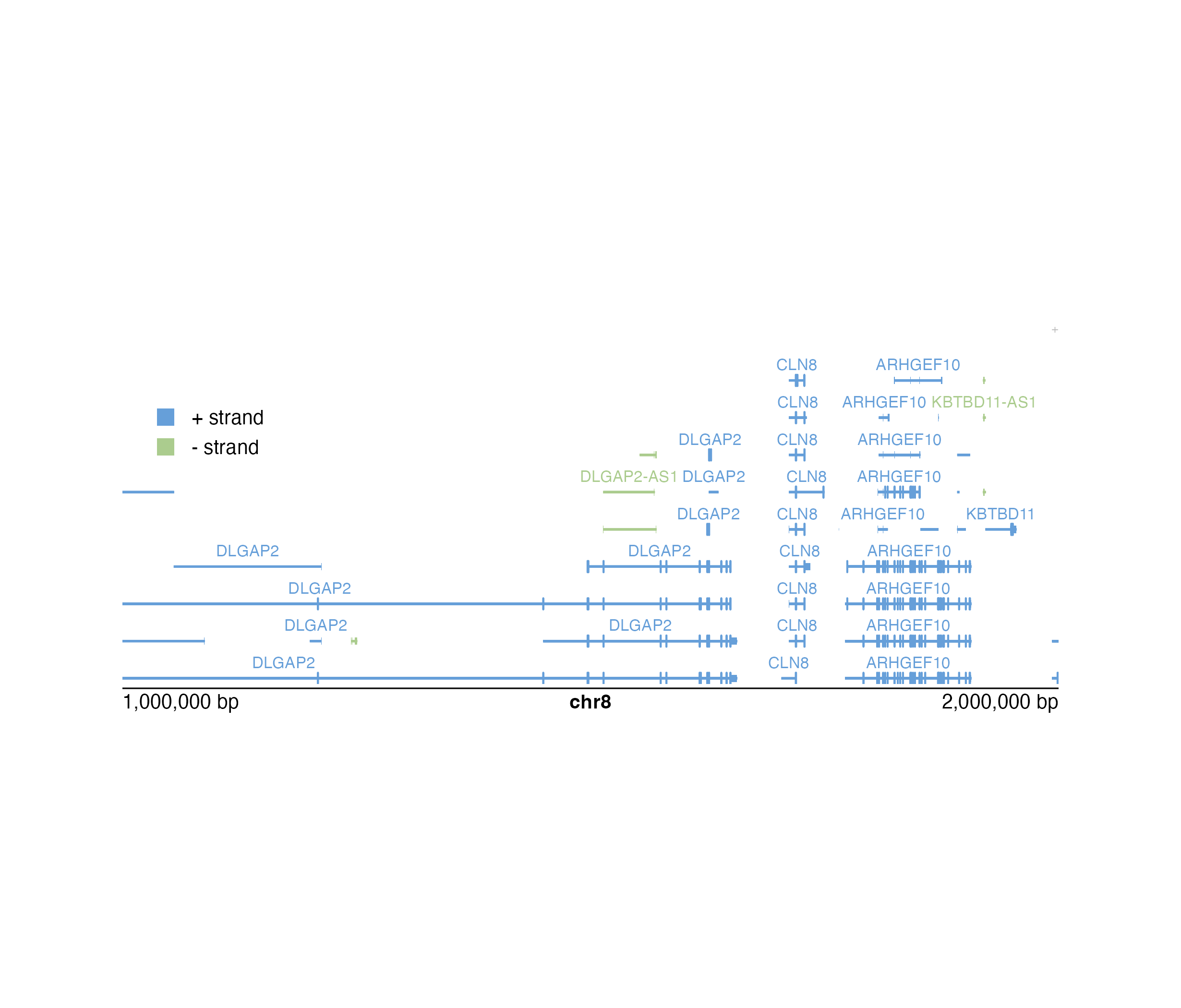Plot gene transcripts in a pileup style for a single chromosome

plotTranscripts(
chrom,
chromstart = NULL,
chromend = NULL,
assembly = "hg38",
fill = c("#669fd9", "#abcc8e"),
colorbyStrand = TRUE,
strandSplit = FALSE,
boxHeight = unit(2, "mm"),
spaceWidth = 0.02,
spaceHeight = 0.3,
limitLabel = TRUE,
fontsize = 8,
labels = "transcript",
stroke = 0.1,
bg = NA,
x = NULL,
y = NULL,
width = NULL,
height = NULL,
just = c("left", "top"),
default.units = "inches",
draw = TRUE,
params = NULL
)

## Arguments

chrom

Chromosome of region to be plotted, as a string.

chromstart

Integer start position on chromosome to be plotted.

chromend

Integer end position on chromosome to be plotted.

assembly

Default genome assembly as a string or a assembly object. Default value is assembly = "hg38".

fill

Character value(s) as a single value or vector specifying fill colors of transcripts. Default value is fill = c("#669fd9", "#abcc8e").

colorbyStrand

A logical value indicating whether to color plus and minus strands by the first two colors in a fill vector, where plus strand transcripts will be colored by the first fill color and minus strand transcripts will be colored by the second fill color. Default value is colorbyStrand = TRUE.

strandSplit

A logical value indicating whether plus and minus-stranded transcripts should be separated, with plus strand transcripts plotted above the x-axis and minus strand transcripts plotted below the x-axis. Default value is strandSplit = FALSE.

boxHeight

A numeric or unit object specifying height of transcripts. Default value is boxHeight = unit(2, "mm").

spaceWidth

A numeric value specifying the width of minimum spacing between transcripts, as a fraction of the plot's genomic range. Default value is spaceWidth = 0.02.

spaceHeight

A numeric value specifying the height of spacing between transcripts on different rows, as a fraction of boxHeight. Default value is spaceHeight = 0.3.

limitLabel

A logical value indicating whether to draw a "+" when not all elements can be plotted in the plotting space. Default value is limitLabel = TRUE.

fontsize

A numeric specifying text fontsize in points. Default value is fontsize = 8.

labels

A character value describing the format of transcript text labels. Default value is labels = "trancript". Options are:

• NULL: No labels.

• "transcript": Transcript name labels.

• "gene": Gene name labels.

• "both": Combined transcript and gene name labels with the format "gene name:transcript name".

stroke

A numeric value indicating the stroke width for transcript body outlines. Default value is stroke = 0.1.

bg

Character value indicating background color. Default value is bg = NA.

x

A numeric or unit object specifying transcript plot x-location.

y

A numeric, unit object, or character containing a "b" combined with a numeric value specifying transcript plot y-location. The character value will place the transcript plot y relative to the bottom of the most recently plotted plot according to the units of the plotgardener page.

width

A numeric or unit object specifying transcript plot width.

height

A numeric or unit object specifying transcript plot height.

just

Justification of transcript plot relative to its (x, y) location. If there are two values, the first value specifies horizontal justification and the second value specifies vertical justification. Possible string values are: "left", "right", "centre", "center", "bottom", and "top". Default value is just = c("left", "top").

default.units

A string indicating the default units to use if x, y, width, or height are only given as numerics. Default value is default.units = "inches".

draw

A logical value indicating whether graphics output should be produced. Default value is draw = TRUE.

params

An optional pgParams object containing relevant function parameters.

## Value

Returns a transcripts object containing relevant genomic region, placement, and grob information.

## Details

A transcripts plot can be placed on a plotgardener coordinate page by providing plot placement parameters:

plotTranscripts(chrom, chromstart = NULL, chromend = NULL,
x, y, width, height, just = c("left", "top"),
default.units = "inches")

This function can also be used to quickly plot an unannotated transcripts plot by ignoring plot placement parameters:

plotTranscripts(chrom, chromstart = NULL, chromend = NULL)

Genomic annotation information is acquired through TxDb and OrgDb-class packages, as determined through the assembly parameter.

## Examples

## Load hg19 genomic annotation packages
library("TxDb.Hsapiens.UCSC.hg19.knownGene")
library("org.Hs.eg.db")

## Create page
pageCreate(width = 7.5, height = 3.5, default.units = "inches")

## Plot and place transcripts
plotTranscripts(
chrom = "chr8", chromstart = 1000000, chromend = 2000000,
assembly = "hg19", labels = "gene",
x = 0.5, y = 0.5, width = 6.5, height = 2.5,
just = c("left", "top"), default.units = "inches"
)
#> transcripts[transcripts1]

## Plot genome label
plotGenomeLabel(
chrom = "chr8", chromstart = 1000000, chromend = 2000000,
assembly = "hg19",
x = 0.5, y = 3.03, length = 6.5, default.units = "inches"
)
#> genomeLabel[genomeLabel1]

## Plot a legend
plotLegend(
legend = c("+ strand", "- strand"),
fill = c("#669fd9", "#abcc8e"), border = FALSE,
x = 0.5, y = 1, width = 1, height = 0.5,
just = c("left", "top")
)
#> legend[legend1]## Hide page guides
pageGuideHide()